Pythagoras: two favourite proofs – Degree of Freedom

# Pythagoras: two favourite proofs

Recently in class when I asked students to name five greatest mathematicians (Newton, Leibniz, Euler, Gauss, Poincaré, Hilbert — and yes, I do know how to count), someone bravely shouted Pythagoras. Pythagoras gets points for being a vegetarian, if he indeed was a vegetarian, and for having a golden thigh, but if his claim to mathematical fame rests on having proved the Pythagoras theorem, then it should be more widely known that he did not, and that his proof is known from earlier Babylonian sources.

There are many proofs of the Pythagoras theorem, which as you know says that in a right angle triangle with hypotenuseand sides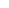andwe necessarily have that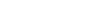My favourite simple proof is given below: just work out the length of the side of the small square in the middle, and get the theorem from the fact that the area of the big square must equal the area of the small square together with the four triangles.But I also have a favourite complicated proof. This one comes from a book by Gregory Barenblatt (Scaling, CUP, Cambridge 2003) which I recommend. This proof is guaranteed to break the ice at parties. Why should one bother with a complicated proof of a simple fact? Because it introduces in a reasonably straightforward way a very useful idea which can be used for harder facts.

First let us introduce some notation. We will use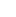to denote the units of a quantity. So for example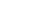is kg, or some multiple thereof.

Let us see one application of thinking in units, or as it is put more frequently, “dimensionally”. Consider a pendulum with a bob of mass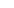, and arm of length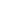. Clearly, it swings about because there is the force of gravity, so the acceleration of free fall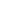must also enter the picture. I claim that the period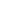of oscillations of the pendulum must be proportional to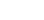. How do I know?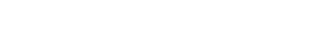.

So the only way to get seconds out of the ingredients of the problem (,,) is to disregard the mass of the bob (there is nothing that will cancel out kilograms!) and take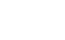because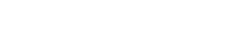.

This type of analysis, “dimensional analysis”, is powerful stuff. Let us see how to prove Pythagoras’ theorem by dimensional analysis.

First of all note that there are many ways of uniquely specifying a right-angle triangle. For example, it can be specified by the two perpendicular sides,and. A better way to do it, is to specify it by the hypotenuseand the angle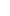at its foot (see Figure 2).Now let us consider this triangle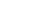. Its area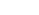is clearly a function of the hypotenuse and the angle,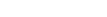.

But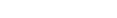, while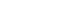andhas no units. (This perhaps will come as a surprise, but if you think about it, you specify angles by ratios of length, so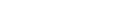. If you are unsure, think about any trigonometric function!) To get the units to match, we must have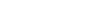,

where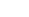is some irrelevantly complicated function of the angle which you are welcome to compute at leisure later. Note that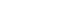.

If we now drop a perpendicular from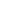onto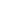, by the same argument as before we have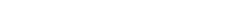.

But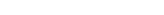.

So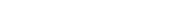.

So now canceland you have Pythagoras’ theorem.

(MG)